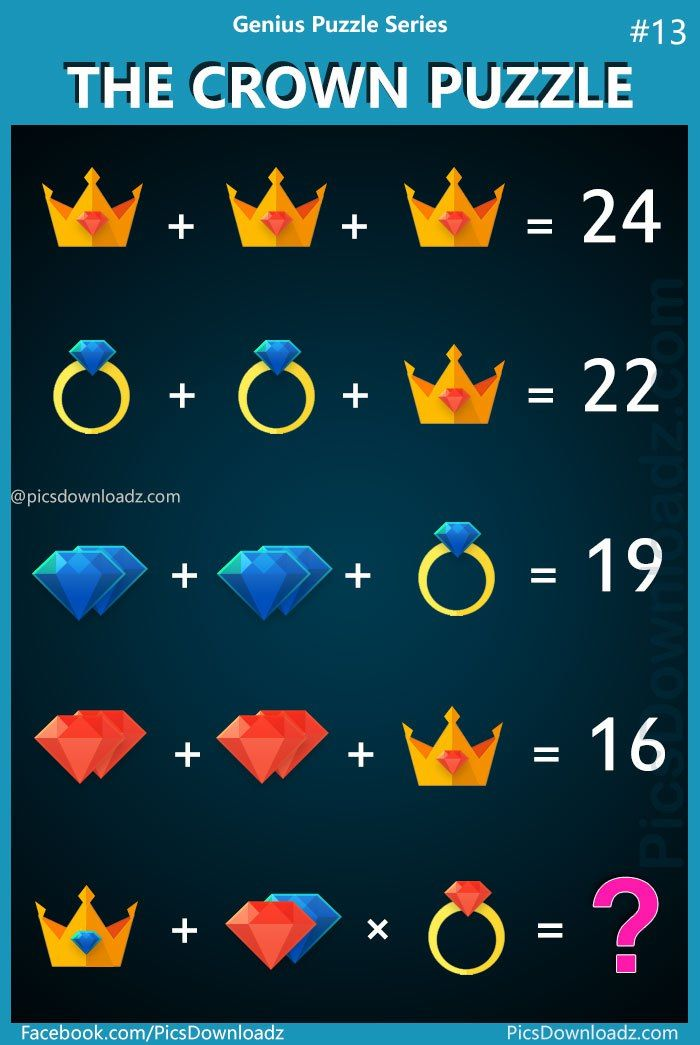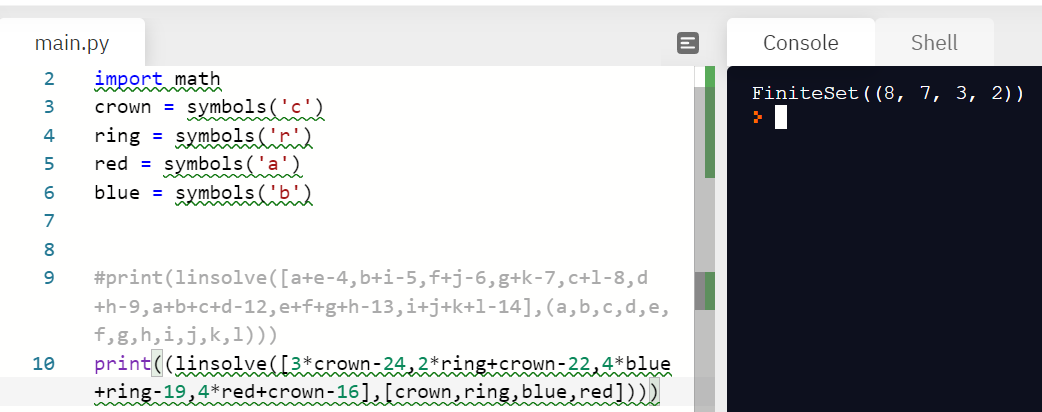# The Crown Puzzle

Okay, I found this problem on the internet, so its obviously not original, but its really tricky, and I liked it a lot, even though its pretty simple. Here it is -So, I will be posting the solution below shortly. If you got this right in the first try and didn't get confused, share it in the comments. If you have a solution that is different than what I have given, please share that too. That's all folks...Note by A Former Brilliant Member
9 months ago

This discussion board is a place to discuss our Daily Challenges and the math and science related to those challenges. Explanations are more than just a solution — they should explain the steps and thinking strategies that you used to obtain the solution. Comments should further the discussion of math and science.

When posting on Brilliant:

• Use the emojis to react to an explanation, whether you're congratulating a job well done , or just really confused .
• Ask specific questions about the challenge or the steps in somebody's explanation. Well-posed questions can add a lot to the discussion, but posting "I don't understand!" doesn't help anyone.
• Try to contribute something new to the discussion, whether it is an extension, generalization or other idea related to the challenge.

MarkdownAppears as
*italics* or _italics_ italics
**bold** or __bold__ bold
- bulleted- list
• bulleted
• list
1. numbered2. list
1. numbered
2. list
Note: you must add a full line of space before and after lists for them to show up correctly
paragraph 1paragraph 2

paragraph 1

paragraph 2

[example link](https://brilliant.org)example link
> This is a quote
This is a quote
    # I indented these lines
# 4 spaces, and now they show
# up as a code block.

print "hello world"
# I indented these lines
# 4 spaces, and now they show
# up as a code block.

print "hello world"
MathAppears as
Remember to wrap math in $$ ... $$ or $ ... $ to ensure proper formatting.
2 \times 3 $2 \times 3$
2^{34} $2^{34}$
a_{i-1} $a_{i-1}$
\frac{2}{3} $\frac{2}{3}$
\sqrt{2} $\sqrt{2}$
\sum_{i=1}^3 $\sum_{i=1}^3$
\sin \theta $\sin \theta$
\boxed{123} $\boxed{123}$

Sort by:

$Key:$ $\color{#3D99F6}Blue gem=B$ $\color{#D61F06}Red gem=R$ $\color{#CEBB00}Crown=C$ $\color{#EC7300}Ring=O$ (to make the explanation simpler, I got rid of the + sign so for example of a crown with red gem, I use CR instead, and it's not multiplication.)

Let's list the information: $\color{#CEBB00} \text{C} \color{#D61F06} \text{R} \color{#333333} \text{ + } \color{#CEBB00} \text{C} \color{#D61F06} \text{R} \color{#333333} \text{ + } \color{#CEBB00} \text{C} \color{#D61F06} \text{R} \color{#333333} \text{ = 24}$

$\color{#3D99F6} \text{B} \color{#EC7300} \text{O} \color{#333333} \text{ + } \color{#3D99F6} \text{B} \color{#EC7300} \text{O} \color{#333333} \text{ + } \color{#CEBB00} \text{C} \color{#D61F06} \text{R} \color{#333333} \text{ = 22}$

$\color{#3D99F6} \text{BB} \color{#333333} \text{ + } \color{#3D99F6} \text{BB} \color{#333333} \text{ + } \color{#3D99F6} \text{B} \color{#EC7300} \text{O} \color{#333333} \text{ = 19}$

$\color{#D61F06} \text{RR} \color{#333333} \text{ + } \color{#D61F06} \text{RR} \color{#333333} \text{ + } \color{#CEBB00} \text{C} \color{#D61F06} \text{R} \color{#333333} \text{ = 16}$

1. For the first line we already know the $\color{#CEBB00} \text{C} \color{#D61F06} \text{R} \color{#333333} = 24 \div 3 = 8$, so $\color{#CEBB00} \text{C} \color{#D61F06} \text{R} \color{#333333} \text{ = 8}$

2. $22 - \color{#3D99F6} \text{B} \color{#EC7300} \text{O} \color{#333333} - \color{#3D99F6} \text{B} \color{#EC7300} \text{O} \color{#333333} - \color{#CEBB00} \text{C} \color{#D61F06} \text{R}$ $= 22 - 2\color{#3D99F6} \text{B} \color{#EC7300} \text{O} \color{#333333} - 8$ $= 14 \div 2$ $\color{#3D99F6} \text{B} \color{#EC7300} \text{O} \color{#333333} \text{ = 7}$

3. $19 - 7 = 2BB$ $12 \div 4 = B$ $B = 3$

4. $16 - 8 = 2RR$ $8 \div 4 = R$ $R =2$

Now we get all the information, Let's summarise them:

$CR = 8$ $BO = 7$ $B = 3$ $R = 2$ $\text {and we can tell}$ $C = 6$ $O = 4$

Now, for the solution, $CB + RB \times RO$ $= 9 + 5 \times 6$ $= 39$

- 9 months ago

You are the first to actually get it right! Lam got it wrong

lol I experienced this kind of problem before, but they are harder to guess. Like one of the figures is a shirt with a whistle(barely noticeable), and at the end it ask how much was a shirt.

- 9 months ago

lol yeah, even I didn't notice before I got to the last line, then it became easy :)

plz upvote.

- 9 months ago

Done!

That last multiplicative is sneaky sneaky...

- 7 months, 3 weeks ago

lol yea

- 7 months, 3 weeks ago

yea, i missed that, otherwise i found this problem rather simple, and was shocked when i got the answer wrong :)

- 7 months, 2 weeks ago

I did notice that, but I didn't appreciate the fact that I had to do it all over again. :/

- 7 months, 2 weeks ago

@Percy Jackson

$3RC=24\Rightarrow RC=\frac{24}{3}=8\\ 2BR+RC=22\Rightarrow BR=\frac{22-8}{2}=7\\ 4BD+BR=19\Rightarrow BD=\frac{19-7}{4}=3\\ 4RD+RC=16\Rightarrow RD=\frac{16-8}{4}=2, BC=8+(3-2)=9, RR=7-(3-2)=6\\ BC+(RD+BD)×RR=9+(2+3)×6=\boxed{39}$

- 9 months ago

lol you just changed it.

- 9 months ago

lol

This is very easy. One crown is 8 because 8x3=24, and one ring is 7 because 22-8=14 and 14/2=7. 19-7=12, so 1 blue diamond=3, since 12/4=3. One crown=8, so one red diamond=2, since 16-8=8 and 8/4=2. Now we can see that one crown without a diamond is 6, since 8-2=6. Also, one ring without a diamond is 4, since 7-3=4. So, one crown with a blue diamond is 9, since 6+3=9. The gems add up to 5, since 3+2=5. One ring with a red diamond is 6, since 4+2=6.

Multiply them together to get 39, since 9 + (5 x 6) = 39.

- 6 months, 4 weeks ago

You forgot PEDMAS/PODMAS/BODMAS/BEDMAS Granger...Multiplication is done before addition. So its $9 + (5 \times 6)$, not $(9+5) \times 6$ @Hermione Granger

- 6 months, 4 weeks ago

- 6 months, 4 weeks ago

I'll change my comment now

- 6 months, 4 weeks ago

Good :)

- 6 months, 4 weeks ago

I used code to solve this question:Now that we know the four important values are, we can solve the actual question.

(8-6+4)*(3+2)+(8-2+3), which corresponds to:

(RING-BLUE+RED)*(RED JEWEL + BLUE JEWEL) + (CROWN-RED+BLUE)

- 6 months, 2 weeks ago

Hmmm, repl.it @Anonymous1 Assassin?

- 6 months, 2 weeks ago

yep

- 6 months, 1 week ago

- 6 months ago

Oh, k :)

bruh lol. ;)

- 6 months ago

I agree that the answer is 39 I found it out this way

RC+RC+RC =RC×3 = 24

3RC = 24

RC÷3 = 24÷3

RC =8

And that crown has a Red gem

Then

BRi+BRi+ C=22

BRi+BRi+8=22

2BRi+8-8=22-8

2BRi=14

2BRi÷2=14÷2

BRi=7

That ring has a blue gem.

2B +2B + BRi =19

4B+7=19

4B+7-7=19-7

4B=12

4B÷4=12÷4

B=3

That is a blue gem

R+R+RC = 16

2R+2R+8-8=16-8

4R=8

4R÷4=8÷4

R=2

That's a red gem

Meaning that the value of a crown with out a red gem is

The value of a crown with a red gem minus the value of a red gem

RC-R=C

8-2=6

C=6

Now a ring with out a gem

BRi-B=Ri

7-3=4

Ri=4

Ri=4 C=6 B=3 R=2

NOOOWW!! The equation

BC+RB×RRi=??

(3+6)+(2+3)×(2+4)

9+5×6

BODMAS

9+(5×6)

9+30

= 39

- 6 months, 1 week ago

wait wa?

- 6 months, 1 week ago

I have added more space, Now do you understand it ?

- 5 months, 3 weeks ago

i cant believed i got trolled by the button

- 9 months ago

It isn't troll, its incomplete

bruh

- 9 months ago

just checked it again... still a troll

- 9 months ago

Lol so true @Percy Jackson

- 9 months ago

Ya, I trolled you guys, yet gave you the solution, it is do complete now....

hahaha, @NSCS 747 you never know what is inside the buttons!

- 9 months ago